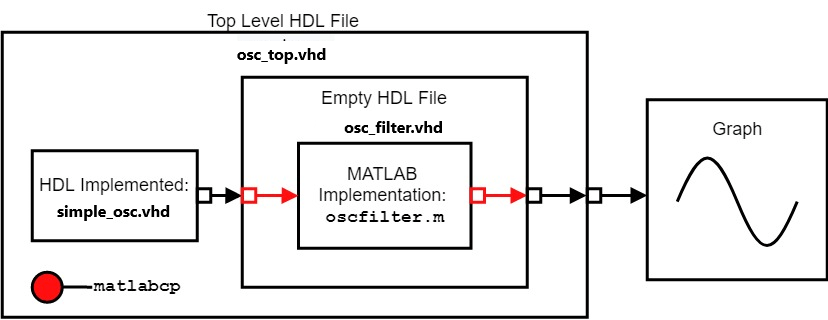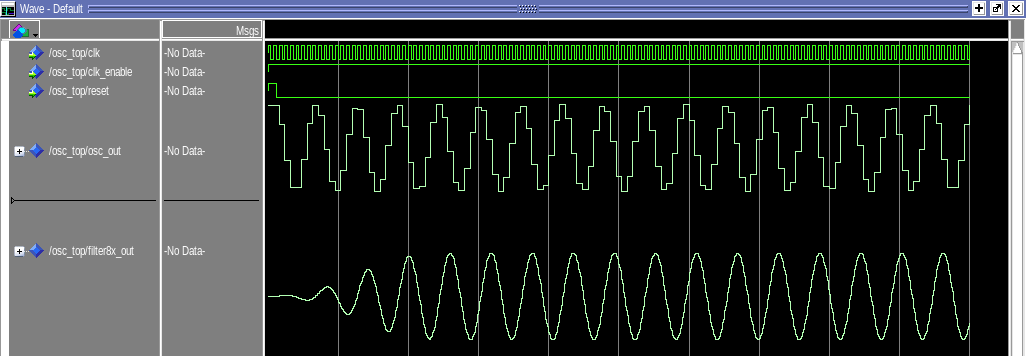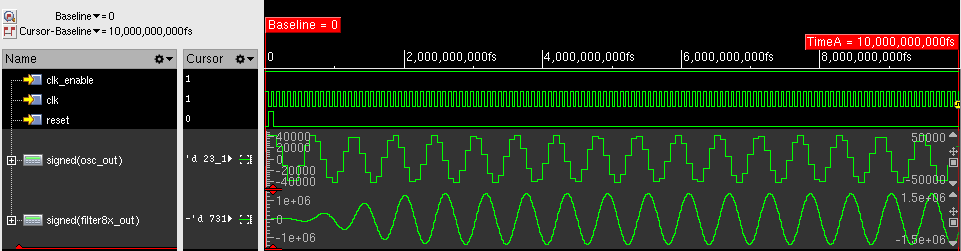# Implement Filter Component of Oscillator in MATLAB

This example shows how to implement a filter component for an HDL project in Mentor Graphics® ModelSim®/Questasim® or Cadence® Xcelium® by using MATLAB® the HDL Verifier™ function `matlabcp`. Instead of using HDL to model a complex component for use in an HDL project, you can use `matlabcp` to implement the component instead. `matlabcp` needs a MATLAB function modeling the component behavior, along with an empty HDL component for input and output ports declared. These files are necessary as `matlabcp` uses the empty component as a shell for communication between MATLAB and the HDL simulator, while the MATLAB function provides the functionality. Using `matlabcp` when verifying a single component allows MATLAB to simulate the various parts of the system, saving time and effort needed to create HDL code for testing. This approach shifts the focus onto generating tests and testing the component in question, creating a more efficient verification workflow. For information on creating the necessary files for `matlabcp`, see Create a MATLAB Component Function.

In this example, the project compiles an oscillator in VHDL® and defines the filter component using MATLAB, before running an HDL simulation. The oscillator is a simple fixed-point sine wave generator written in HDL, and feeds a lowpass filter. This lowpass filter is a 255th order (256-tap) filter with 8x oversampling that is implemented using the polyphase technique in MATLAB. The filter inputs and outputs are carried by `matlabcp` between the HDL simulator and MATLAB, with the results displayed using the simulator waveform viewer. By using simulator waveform viewer to display the results, this example demonstrates that `matlabcp` can seamlessly integrate a component designed in MATLAB into a larger HDL workflow without requiring MATLAB in the later steps. This diagram shows the overall flow of this cosimulation process.This example considers the point of view of an HDL developer, so the main emphasis of this example will be on using the HDL simulator and command-line terminal to perform testing, and using MATLAB to implement some of the project components.

### Overview of Design Files and Script Files

This example uses two types of files: design files and script files.

Design Files

• The file `simple_osc.vhd` contains the simple sinusoidal oscillator designed using VHDL.

• The file `osc_filter.vhd` is the empty component that is used for `matlabcp` to receive the component inputs and transmit the component outputs.

• The file `osc_top.vhd` contains the top-level model of the oscillator and lowpass filter.

• The file `oscfilter.m` contains the behavioral implementation of the filter, implemented in MATLAB.

Script Files

• The file `qcommands_osc_w.tcl` contains the commands that are sent into ModelSim/Questasim on Windows® for cosimulation to occur.

• The file `qcommands_osc_l.tcl` contains the commands that are sent into ModelSim/Questasim on Linux® for cosimulation to occur.

• The file `xcelium_osc.tcl` contains the commands for opening the Xcelium simulator so that MATLAB can communicate with the simulator.

• The file `xmcommands_osc.tcl` contains the commands that are sent into Xcelium for cosimulation to occur.

Additionally, this example requires access to ModelSim/Questasim or Xcelium and MATLAB from the terminal. You must include these products on the system path.

### Adjust the Script Files

This example uses the provided script files to link MATLAB and the HDL simulator. The script uses two commands: a command that invokes the HDL simulator and a command from the MATLAB shared library which creates the connection for cosimulation. To run this example, you need to change the command that invokes the HDL simulator, as the default command relies on the absolute path to the MATLAB shared library. Adjust the script files based on the simulator and operating system.

ModelSim/Questasim:

• Windows: The figure shows lines 3 and 4 of `qcommands_osc_w.tcl`. These lines contain the commands necessary for cosimulation with Modelsim/Questasim on Windows. Line 3 is the call to open ModelSim/Questasim, where the `-foreign` argument supplies the path to the MATLAB shared library, such that Modelsim/Questasim loads in the shared library. Line 4 is the call to a function specified in the shared library that connects the two programs together.For this example to work, you must change line 3 in `qcommands_osc_w.tcl`. Swap `matlabroot` with the install location of MATLAB, so that the shared library can be located and loaded in.

• Linux: The figure below shows lines 3 and 4 of `qcommands_osc_l.tcl`. These lines contain the commands necessary for cosimulation with Modelsim/Questasim on Linux. Line 3 is the call to open ModelSim/Questasim, where the `-foreign` argument supplies the path to the MATLAB shared library, such that Modelsim/Questasim loads in the shared library. Line 4 is the call to a function specified in the shared library that connects the two programs together.For this example to work, you must change line 3 in `qcommands_osc_l.tcl`. Swap `matlabroot` with the install location of MATLAB, so that the shared library can be located and loaded in.

Xcelium

The figure shows lines 3--6 of `xcelium_osc.tcl`, which contain the command that opens the Xcelium simulator. Line 4 tells Xcelium to run a command from the shared library to link the two programs. Line 6 tells Xcelium to load the MATLAB shared library.For this example to run you must change `xcelium_osc.tcl` on line 6. Here you need to replace `matlabroot` with the installation location of MATLAB so that the shared library can be located and loaded in.

### Run Cosimulation

To start cosimulation, open MATLAB and run the HDL Link MATLAB server for communication between the HDL simulator and MATLAB. To complete these actions, use these commands based on operating system.

• Windows: `matlab -nodesktop -r "hdldaemon"`

• Linux: `xterm -e "matlab -nodesktop -r "hdldaemon"" &`

This opens MATLAB in a separate terminal window, in headless mode, and starts the HDL Link MATLAB server. The terminal that is running MATLAB displays this message.

`"HDLDaemon shared memory server is running with 0 connections"`

After this message appears, start the cosimulation by entering the applicable command (based on the simulator and operating system) into a system terminal.

ModelSim/Questasim

• Windows: `vsim -do qcommands_osc_w.tcl`

• Linux: `vsim -do qcommands_osc_l.tcl`

Xcelium

• Linux: `tclsh xcelium_osc.tcl`

After you enter the respective commands, cosimulation starts and produces these plots in the respective HDL simulator. Your plots might appear different right after cosimulation, but zooming to encompass the entire time axis can yield similar looking plots.

ModelSim/QuestasimXcelium### Summary

This example shows how to use MATLAB as a substitute for a major component in the HDL project, replacing the HDL implementation of the lowpass filter. The file `osc_filter.vhd` is a placeholder in the system to feed the inputs into the MATLAB function and send the outputs from the MATLAB function into the rest of the project. The implementation of the filter and process of filtering the input to produce the output is completely driven by the MATLAB function.

You can use MATLAB to replace various components in the HDL simulation project with behavioral implementations in MATLAB, less time is needed to prepare the HDL project. This approach shifts the focus onto testing and verification of the component in question, instead of spending time verifying and creating the other components of the project.